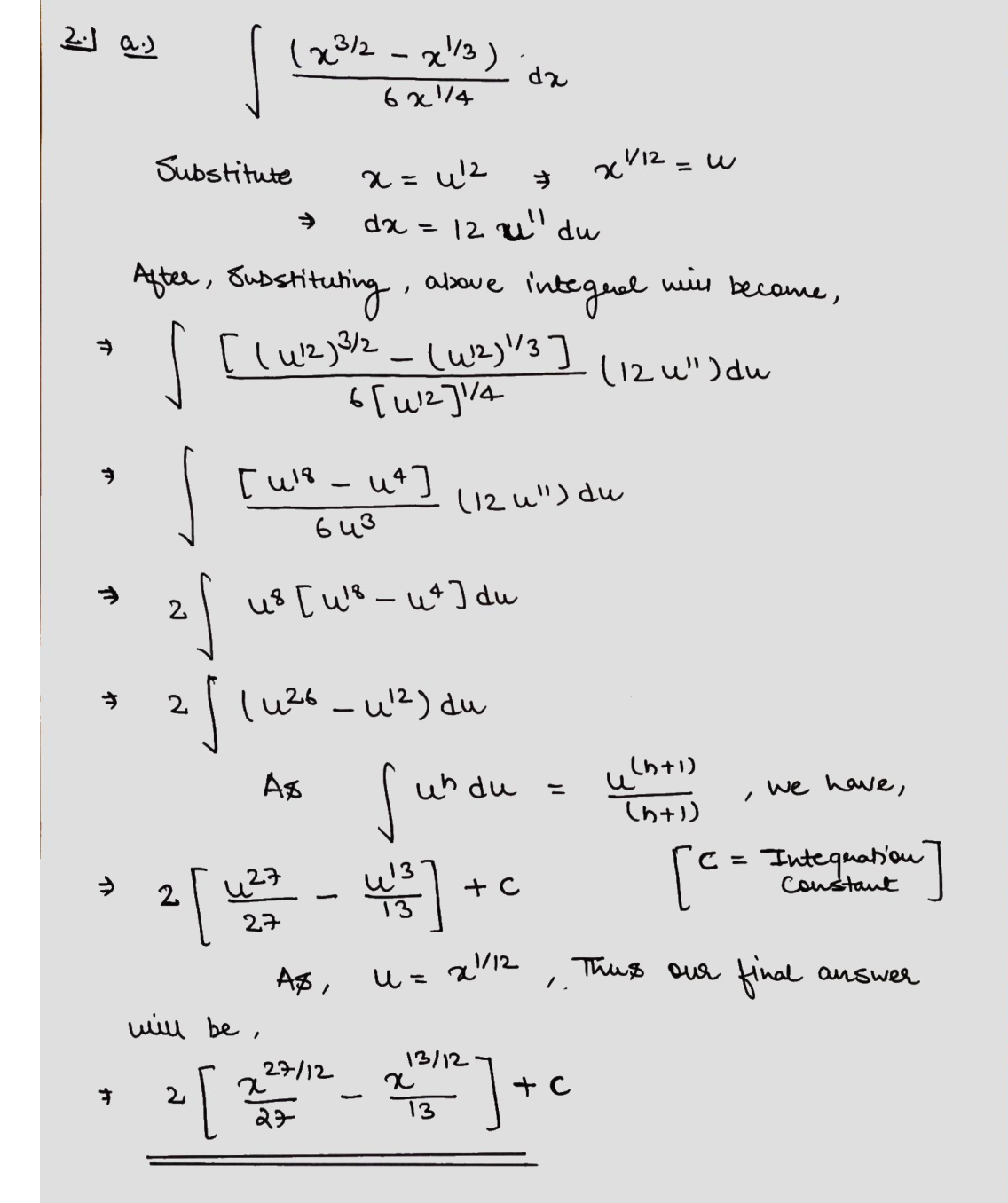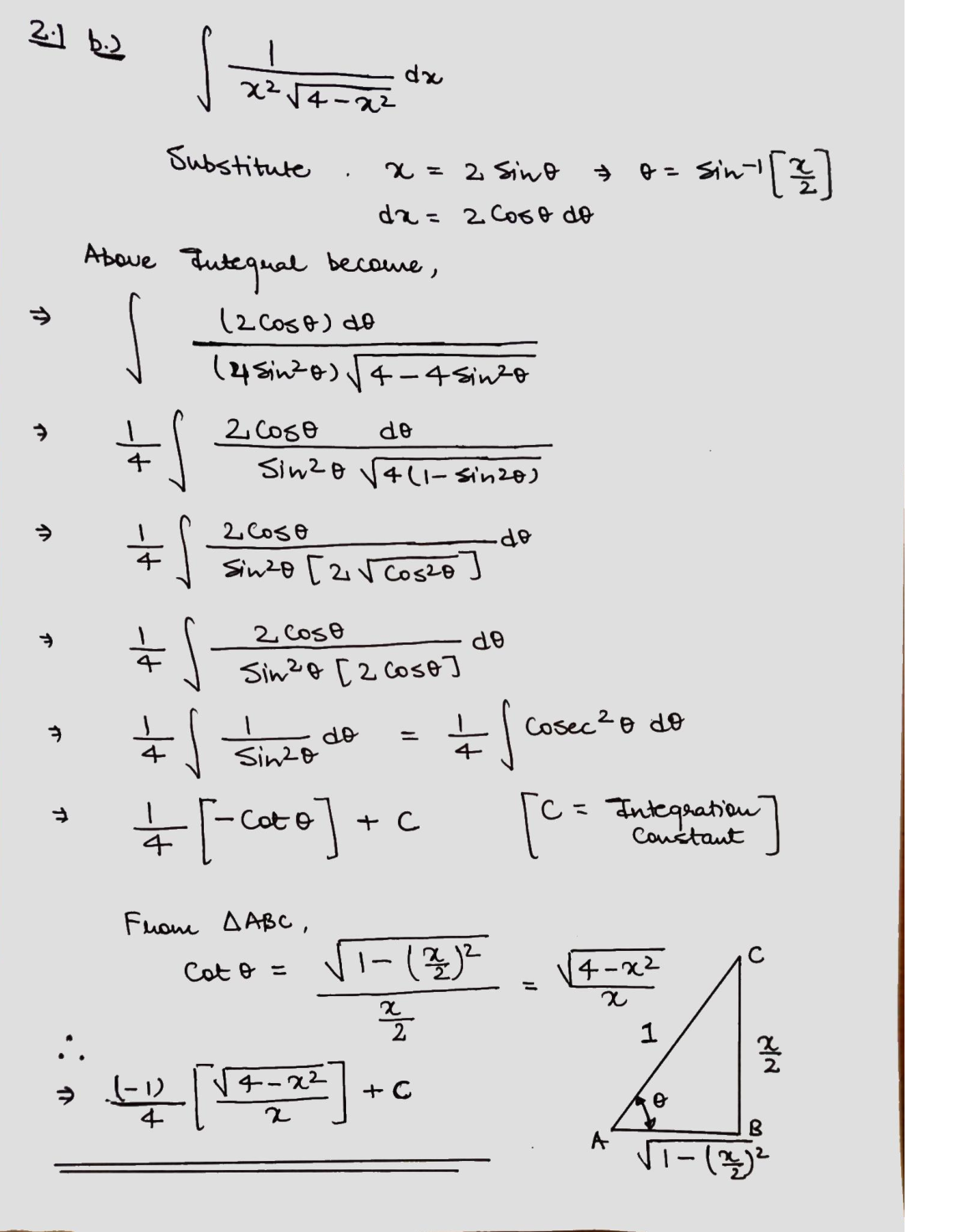Question

Integration

2) Evaluate the following integrals by algebraic or trigonometric substitutions.

\text { a) } \int \frac{\left(x^{3 / 2}-x^{1 / 3}\right)}{6 x^{1 / 4}} d x

\text { b) } \int \frac{1}{x^{2} \sqrt{4-x^{2}}} d xVerified### Question 42010Integration

Convert the equation into spherical coordinates.
\rho=18 \sec (\varphi)
\rho=18 \sin (\varphi)
x^{2}+y^{2}+(z-9)^{2}=81
\rho=\sqrt{18}
\rho=18 \cos (\varphi)

### Question 42009Integration

\left(\sqrt{26}, \frac{\pi}{6}, \frac{\pi}{3}\right)
Convert the spherical point (p, o, 0) into rectangular coordinates.

### Question 42008Integration

Convert the spherical point (p, q,0) into rectangular coordinates.

### Question 42007Integration

Set up and evaluate the indicated triple integral in the appropriate coordinate system. Enter an exactanswer. Do not use a decimal approximation.
\iiint_{Q} z d V, \text { where } Q \text { is the region between } z=\sqrt{x^{2}+y^{2}} \text { and } z=\sqrt{16-x^{2}-y^{2}}
\iiint_{Q} z d V=

### Question 42006Integration

After set up, evaluate the indicated triple integral in the appropriate coordinate system. Enter an exactanswer. Do not use a decimal approximation.
\iiint_{Q} z e^{f(x, y)} d V, f(x, y)=\sqrt{x^{2}+y^{2}}, \text { where } Q \text { is the region inside } x^{2}+y^{2}=100, \text { outside } x^{2}+y^{2}=64
and between z=0 and z=5.
\iiint_{Q} z e^{f(x, y)} d V=

### Question 42005Integration

\text { Set up the triple integral } \iiint_{Q} f(x, y, z) d V \text { in cylindrical coordinates. }
Q \text { is the region bounded by } y=36-x^{2}-z^{2} \text { and } y=3
\int_{0}^{6} \int_{3}^{36-r^{2}} \int_{0}^{2 \pi} f(r \cos (\theta), y, r \sin (\theta)) \cdot r d y d r d \theta
\int_{0}^{36} \int_{3}^{36-r^{2}} \int_{0}^{2 \pi} f(r \cos (\theta), y, r \sin (\theta)) \cdot r d y d r d \theta
\int_{0}^{6} \int_{0}^{2 \pi} \int_{3}^{36-r^{2}} f(r \cos (\theta), y, r \sin (\theta)) \cdot r d y d r d \theta
\int_{0}^{2 \pi} \int_{0}^{6} \int_{3}^{36-r^{2}} f(r \cos (\theta), y, r \sin (\theta)) \cdot r d y d r d \theta

### Question 42004Integration

\text { Set up the triple integral } \iiint_{Q} f(x, y, z) d V \text { in cylindrical coordinates. }
Q \text { is the region above } z=\sqrt{x^{2}+y^{2}} \text { and below } z=\sqrt{1352-x^{2}-y^{2}} .
\int_{0}^{2 \pi} \int_{0}^{26} \int_{r}^{\sqrt{1352-r^{2}}} f(r \cos (\theta), r \sin (\theta), z) d z d r d \theta
\int_{0}^{2 \pi} \int_{0}^{26} \int_{r}^{\sqrt{1352-r^{2}}} f(r \cos (\theta), r \sin (\theta), z) \cdot r d z d r d \theta
\int_{0}^{2 \pi} \int_{0}^{676} \int_{r}^{\sqrt{1352-r^{2}}} f(r \cos (\theta), r \sin (\theta), z) d z d r d \theta
\int_{0}^{2 \pi} \int_{0}^{676} \int_{\nu}^{\sqrt{1352-r^{2}}} f(r \cos (\theta), r \sin (\theta), z) \cdot r d z d r d \theta

### Question 42003Integration

Write the given equation in Cylindrical coordinates.
(x-95)^{2}+y^{2}=9,025
r=95 \sin (\theta)
r=95 \cos (\theta)
r=190 \sin (\theta)
r=190 \cos (\theta)

### Question 42002Integration

Write the given equation in cylindrical coordinates.
x^{2}+y^{2}=196

### Question 42001Integration

Find the mass of the solid with density p(x, y, z) and the given shape.
\rho(x, y, z)=41, \text { solid bounded by } z=x^{2}+y^{2} \text { and } z=9
Mass

### Submit query